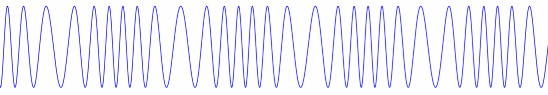# Energy in frequency modulated signals

In an earlier post we proved that if you modulate a cosine carrier by a sine signal you get a signal whose sideband amplitudes are given by Bessel functions. Specifically:When β = 0, we have the unmodulated carrier, cos(2π fct), on both sides. When β is positive but small, J0(β) is near 1, and so the frequency component corresponding to the carrier is only slightly diminished. Also, the sideband amplitudes, the values of Jn(β) for n ≠ 0, are small and decay rapidly as |n| increases. As β increases, the amplitude of the carrier component decreases, the sideband amplitudes increase, and the sidebands decay more slowly.

We can be much more precise: the energy in the modulated signal is the same as the energy in the unmodulated signal. As β increases, more energy transfers to the sidebands, but the total energy stays the same. This conservation of energy result applies to more complex signals than just pure sine waves, but it’s easier to demonstrate in the case of a simple signal.## Proof

To prove the energy stays constant, we show that the sum of the squares of the coefficients of the cosine components is the same for the modulated and unmodulated signal.The unmodulated signal is just cos(2π fct), and so the only coefficient is 1. That means we have to proveThis is a well-known result. For example, it is equation 9.1.76 in Abramowitz and Stegun. We’ll show how to prove it from first principles. We’ll actually prove a more general result, the Newmann-Schläffi addition formula, then show our result follows easily from that.

Whittaker and Watson define the Bessel functions by their generating function:This means that when you expand the expression on the left as a power series in t, whatever is multiplied by tn is Jn(z) by definition. (There are other ways of defining the Bessel functions, but this way leads quickly to what we want to prove.)

We begin by factoring the Bessel generating function applied to zw.Next we expand both sides as power series.and look at the terms involving tn on both sides. On the left this is Jn(zw). On the right, we multiply two power series. We will get a term containing tn whenever we multiply terms tj and tk where j and k sum to n.The equation above is the Newmann-Schläffi addition formula.

### Sum of squared coefficients

To prove that the sum of the squared sideband coefficients is 1,  we apply the addition formula with n = 0, z = β, and w = -β.This proves what we were after:We used a couple facts in the last step that we haven’t discussed. The first was that J0(0) = 1. This follows from the generating function by setting z to 0 and taking the limit as t → 0. The second was that Jm(-β) = Jm(β). You can also see this from the generating function since negating z has the same effect as swapping t and 1/t.